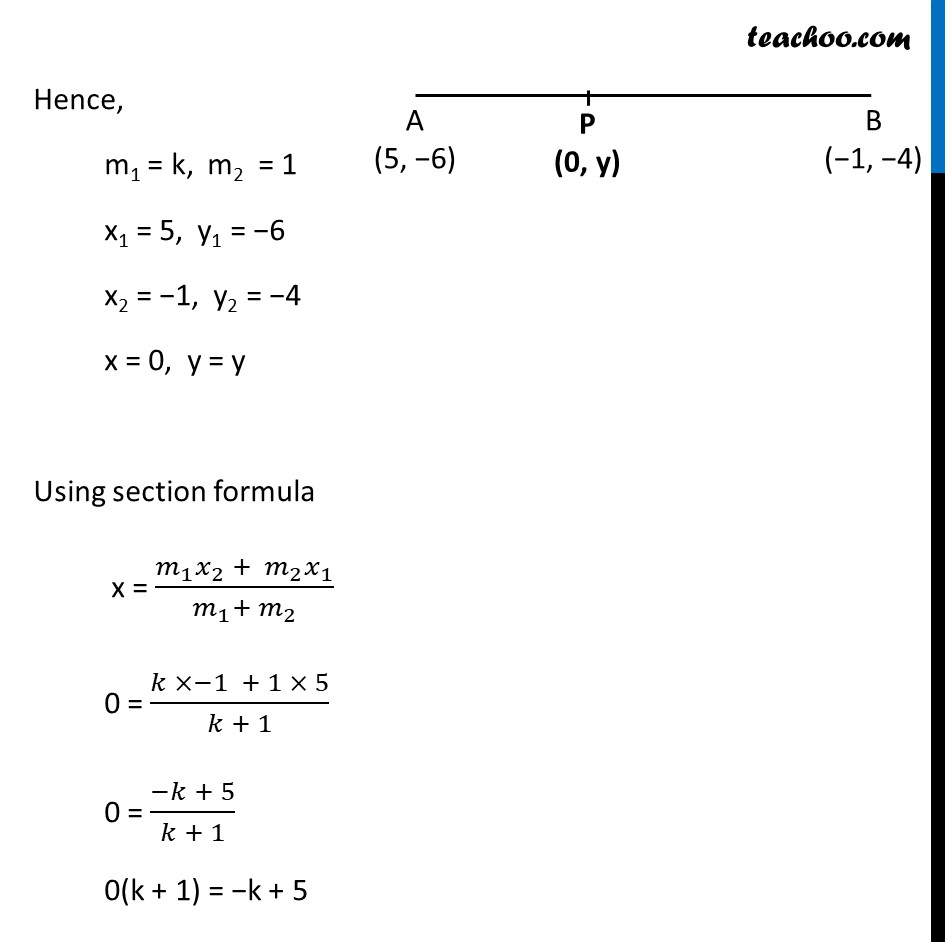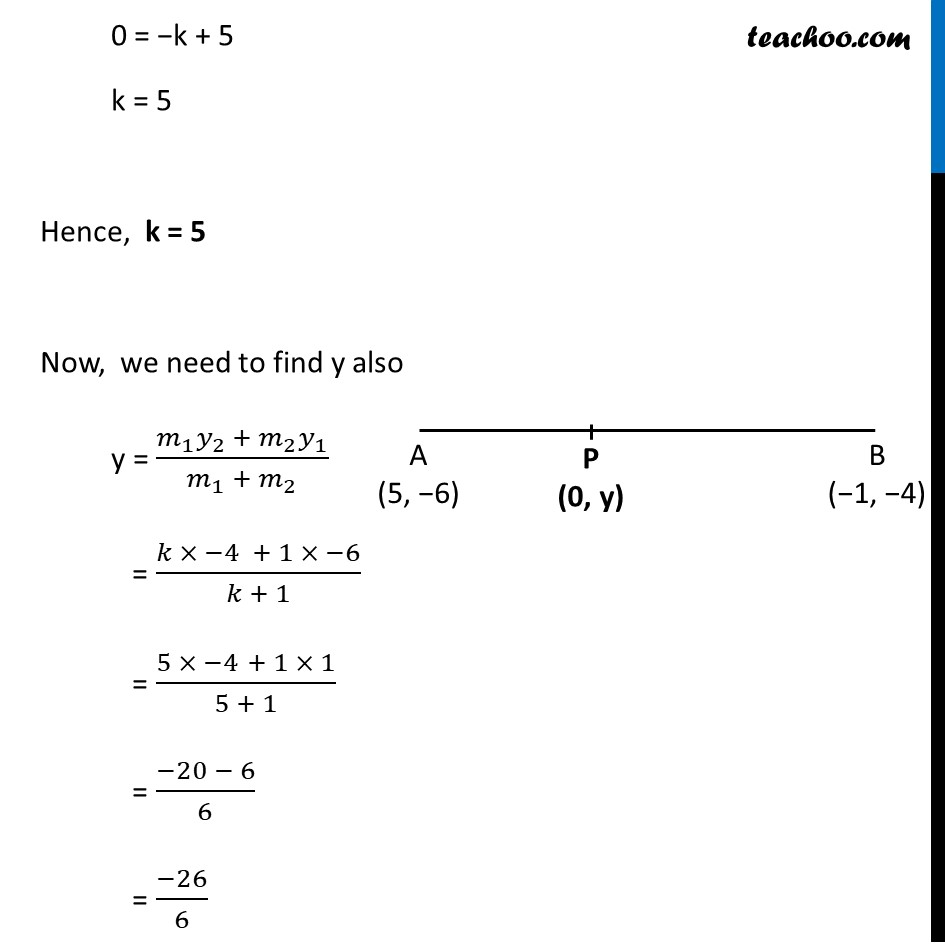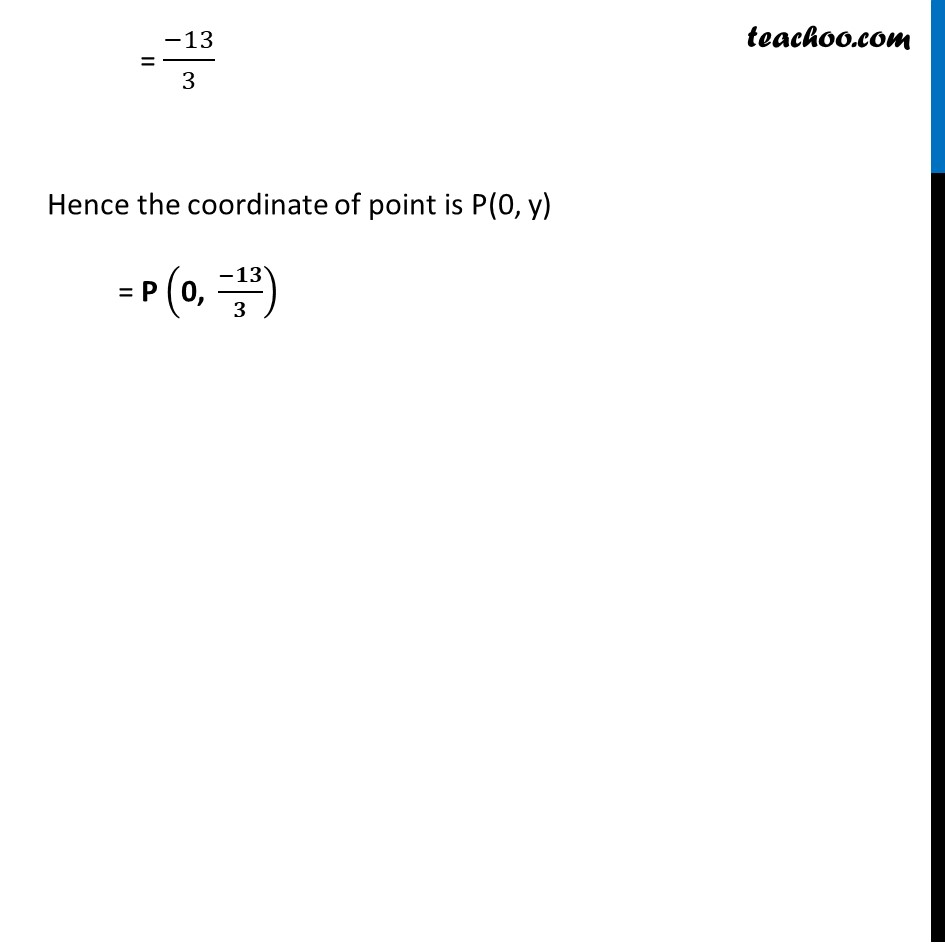Examples

Chapter 7 Class 10 Coordinate Geometry
Serial order wiseLearn in your speed, with individual attention - Teachoo Maths 1-on-1 Class

### Transcript

Example 9 Find the ratio in which the y−axis divides the line segment joining the points (5, – 6) and (–1, – 4). Also find the point of intersection. Let the point be A(5, −6) & B(−1, −4) Let Point P the required required point Since Point P is on y−axis, hence its x coordinate is 0. So, it is of the form P(0, y) Now, we have to find ratio Let ratio be k : 1 Hence, m1 = k, m2 = 1 x1 = 5, y1 = −6 x2 = −1, y2 = −4 x = 0, y = y Using section formula x = (𝑚_1 𝑥_2 + 𝑚_2 𝑥_1)/(𝑚_1+ 𝑚_2 ) 0 = (𝑘 ×−1 + 1 × 5)/(𝑘 + 1) 0 = (−𝑘 + 5)/(𝑘 + 1) 0(k + 1) = −k + 5 0 = −k + 5 k = 5 Hence, k = 5 Now, we need to find y also y = (𝑚_1 𝑦_2 + 𝑚_2 𝑦_1)/(𝑚_1 + 𝑚_2 ) = (𝑘 × −4 + 1 × −6)/(𝑘 + 1) = (5 × −4 + 1 × 1)/(5 + 1) = (−20 − 6)/6 = (−26)/6 = (−13)/3 Hence the coordinate of point is P(0, y) = P ("0, " (−𝟏𝟑)/𝟑)